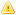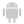Home |Sign in | EnglishHelpWLanguageWLanguage functionsControls, pages and windowsDrawing functionsPrefix syntaxDrawing functions on picLayer variablesDrawPolygonPresentationExampleCoordinatesDrawing with opacity or anti-aliasingRelated examplesSee alsoAlphaBlendBackgroundChangeModeDrawChordDrawLineDrawPointDrawPolygonDrawPolylineDrawRectangleDrawRectangleGradientDrawRoundedRectangleDrawSliceDrawTextDrawTextRTFEndDrawingFillFontHorizontalSymmetryInvertColorPenPixelColorPixelOpacityResizeRotationStartDrawingVerticalSymmetryThis content has been translated automatically. Click here to view the French version.
• Coordinates
• Drawing with opacity or anti-aliasing
WINDEVWEBDEVWINDEV MobileOthers<picLayer variable>.DrawPolygon (Function)
In french: <Variable picCalque>.DessinePolygone
Draws a polygon in an picLayer variable.
Example
MonImageWDPic is WDPic = "Test.gif"
MonCalque is picLayer

// Dessin d'un polygone à 4 côtés dont le
// fond est jaune clair et le bord bleu clair
MonCalque.DrawPolygon(4, 10, 20, 20, 50, 40, 60, 50, 10, LightYellow, LightBlue)
IMG_MonDessin = MonImageWDPic
Syntax

Building a polygon point by point

<picLayer image>.DrawPolygon(<Number of sides> , <X1> , <Y1> , <X2> , <Y2> [, <X3> , <Y3> [... [, <Xn> , <Yn>]]] [, <Background color> [, <Side color>]])
<picLayer image>: picLayer variable
Name of the variable of type picLayer to be used.
<Number of sides>: Integer
Number of sides in the polygon (up to 23 sides). This parameter defines the number of <X>, <Y> pairs to specify in the function.
<X1>: Integer
X-coordinate of first point of the polygon. These coordinates are expressed in pixels.
<Y1>: Integer
Y-coordinate of first point of the polygon. These coordinates are expressed in pixels.
<X2>: Integer
X-coordinate of second point of the polygon. These coordinates are expressed in pixels.
<Y2>: Integer
Y-coordinate of second point of the polygon. These coordinates are expressed in pixels.
<X3>: Integer
X-coordinate of 3rd point of the polygon. These coordinates are expressed in pixels.
<Y3>: Integer
Y-coordinate of 3rd point of the polygon. These coordinates are expressed in pixels.
<Xn>: Optional integer
X coordinate of nth point of the polygon. These coordinates are expressed in pixels.
<Yn>: Optional integer
Y coordinate of nth point of the polygon. These coordinates are expressed in pixels.
<Background color>: Integer or constant (optional)
Polygon background color. This color can correspond to:
If this parameter is not specified, the background color:
<Side color>: Integer or constant (optional)
Color of polygon sides. This color can correspond to:
If this parameter is not specified, the line color:
• corresponds to the Color specified in the last call to the dPen function,
• is identical to the Color used for the Background if the dPen function was not used.

Building a polygon via an array of X and Y-coordinates

<picLayer image>.DrawPolygon(<Array of coordinates> [, <Background color> [, <Side color>]])
<picLayer image>: picLayer variable
Name of the variable of type picLayer to be used.
<Array of coordinates>: Array of integers or array of points
• WLanguage array of integers containing the coordinates of polygon points. The even subscript represent the X-coordinates while the odd subscripts represent the Y-coordinates of points. These coordinates are expressed in pixels.
<Background color>: Integer or constant (optional)
Polygon background color. This color can correspond to:
If this parameter is not specified, the background color:
<Side color>: Integer or constant (optional)
Color of polygon sides. This color can correspond to:
If this parameter is not specified, the line color:
• corresponds to the color specified in the last call to dPen,
• is identical to the Color used for the Background if the dPen function was not used.Versions 26 and laterConstruction of a polygon from an Variable of the Polygon type

<picLayer image>.DrawPolygon(<Polygon> [, <Background color> [, <Side color>]])
<picLayer image>: picLayer variable
Name of the variable of type picLayer to be used.
<Polygon>: Variable type Polygon
Name of the variable of type Polygon describing the different points of the Polygon.
<Background color>: Integer or constant (optional)
Polygon background color. This color can correspond to:
If this parameter is not specified, the background color:
<Side color>: Integer or constant (optional)
Color of polygon sides. This color can correspond to:
If this parameter is not specified, the line color:
• corresponds to the color specified in the last call to dPen,
• is identical to the Color used for the Background if the dPen function was not used.New in version 26Construction of a polygon from an Variable of the Polygon type

<picLayer image>.DrawPolygon(<Polygon> [, <Background color> [, <Side color>]])
<picLayer image>: picLayer variable
Name of the variable of type picLayer to be used.
<Polygon>: Variable type Polygon
Name of the variable of type Polygon describing the different points of the Polygon.
<Background color>: Integer or constant (optional)
Polygon background color. This color can correspond to:
If this parameter is not specified, the background color:
<Side color>: Integer or constant (optional)
Color of polygon sides. This color can correspond to:
If this parameter is not specified, the line color:
• corresponds to the color specified in the last call to dPen,
• is identical to the Color used for the Background if the dPen function was not used.Construction of a polygon from an Variable of the Polygon type

<picLayer image>.DrawPolygon(<Polygon> [, <Background color> [, <Side color>]])
<picLayer image>: picLayer variable
Name of the variable of type picLayer to be used.
<Polygon>: Variable type Polygon
Name of the variable of type Polygon describing the different points of the Polygon.
<Background color>: Integer or constant (optional)
Polygon background color. This color can correspond to:
If this parameter is not specified, the background color:
<Side color>: Integer or constant (optional)
Color of polygon sides. This color can correspond to:
If this parameter is not specified, the line color:
• corresponds to the color specified in the last call to dPen,
• is identical to the Color used for the Background if the dPen function was not used.
Remarks

Coordinates

Drawing a polygon:
MonImage is WDPic
MonCalque is picLayer

MonCalque.DrawPolygon(5, 80, 10, 10, 150, 100, 200, 160, 80, 140, 40)Coordinates are given with respect to the top left corner of the image (coordinates: (0.0)).
Important: There is no need to close the polygon (the coordinates of last point do not have to be equal to the coordinates of first point). The polygon is automatically "closed".
Limits: a polygon can contain up to 100 000 points in WINDEV, WEBDEV and Java, and up to 1000 points in Windows CE.Drawing with opacity or anti-aliasing

When drawing with opacity (<picLayer variable>.StartDrawing with the dWithOpacity constant) or with anti-aliasing (<picLayer variable>.ChangeMode with the drawAntiAliasing constant), the border is drawn above the inside of the polygon: therefore, the line is partly combined with the inside of the polygon. If the line is very thick, it will be displayed in two colors.
Related Examples:Unit examples (WINDEV): The drawing functions [ + ] Using the main drawing functions of WINDEV to:- Initialize an Image control for drawing- Draw simple shapes- Write a text into a drawing- Change the color in a drawing
Business / UI classification: Neutral code
Component: wd270pnt.dll
Minimum version required
• Version 25